# Counting colourings with containers

On the maximum number of integer colourings with forbidden monochromatic sums, Hong Liu, Maryam Sharifzadeh and Katherine Staden

The problem is as follows.  Let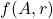be the number of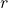-colourings of a subset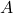of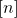with no monochromatic sum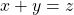.  What is the maximum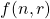ofover all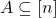?

One possibility is that we taketo be sum-free, so that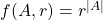.  The maximum size of a sum-free set ofis around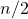, achieved by the set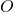of odd numbers and the interval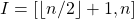, so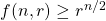.

Another possibility is to choosesum-free sets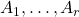and take all colourings of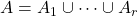such that the elements of colourare contained in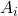.  There are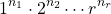such colourings, where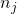is the number of elements in exactly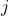of the.  For example, we might take half of theto beand half to be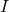.  Then the odd numbers greater thanare in every set, and the evens greater thanand the odds less thanare in half of the sets, so the number of colourings is around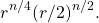For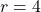this matches the previous lower bound; for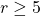it is larger.

It’s easy to see that this construction cannot improve the bound for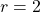: it only provides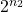good colourings, but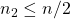as elements contributing to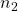are in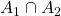, which must be sum-free.

What about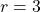?  Now we get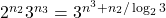good colourings.  We also have that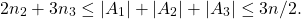But since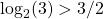we have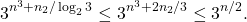Moreover, ifis not tiny then we are some distance off this upper bound, so the only good constructions in this family come from having all thesubstantially agree.

How can we get matching upper bounds?  If there weren’t very many maximal sum-free sets then we could say that every good colouring arises from a construction like this, and there aren’t too many such constructions to consider.  This is too optimistic, but the argument can be patched up using containers.

The container method is a relatively recent addition to the combinatorial toolset.  For this problem the key fact is that there is a set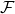of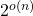subsets ofsuch that

• every sum-free set is contained in some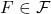,
• eachis close to sum-free.  Combined with a “sum-removal lemma” this means in particular that it has size not much larger than.

We now consider running the above construction with eachan element of.  Since the containers are not themselves sum-free, this will produce some bad colourings.  But because every sum-free set is contained in some element of, every good colouring of a subsetofwill arise in this way.  And since there are most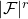choices for the setsthe number of colourings we produce is at most a factorgreater than the biggest single example arising from the construction.

This is the big idea of the paper: it reduces counting colourings to the problem of optimising this one construction.  For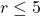the authors are able to solve this new problem, and so the original.